Lecture notes, intro material, textbooks and helpful papers, and related web links, all on aspects of geophysical inverse theory...

Introductory material (by me):
A Conceptual Introduction to Geophysical Inversion. PDF file, presented in the UW Earth & Space Sciences brown bag series. No math in this one, just an overview level talk, basically the graphical version of the primer below.
A Geophysical Inverse Theory Primer. This document (PDF file) is ten pages long, contains no equations, and aims to provide an overview of the main concepts in inverse theory. By giving a summary at a high-level, the goal is to introduce the subject to the new user, and place the different concepts and solution methods in perspective with each other before delving into mathematical details.
An introduction to geophysical inversion, with comparisons to analytical inversion. Powerpoint file, presented on invitation to the UW Math Dept Inverse Problems seminar series, posted here on request of several colleagues. Many more equations than above; you would definitely want to start with the above primer first if you are new to inverse theory.

My Inverse Theory Computational Lab Lecture Notes plus Tutorial Matlab scripts from class:
Used in conjunction with University of Washington's (Seattle) ESS523, Geophysical Inverse Theory, professor Ken Creager.
• LAB #1: A review tutorial script covering Matlab scripting fundamentals and some linear algebra review. This is a long script in which everything is initially commented out. Read and uncomment it bit by bit, running local sections of it to learn key concepts. It ends with an assignment to do a parabolic curve fitting to noisy data.
• LAB #1.5: A tutorial exploring the meaning of covariance and related statistical concepts. As with reviewtut.m above, the idea is to read and uncomment it bit by bit.
• LAB #2: An extension to the curve fitting in lab #1; now we compute statistical measures of the quality of the curve fit we'd found in lab #1.
• LAB #3: Linear inversion of several density profiles of the Earth using the Gram matrix / inner product approach we learned in class and in Parker's book.
• LAB #4: Parameter estimation of earthquake source location given arrival times at known receiver station locations - a weakly nonlinear problem.
• LAB #5: Objective surfaces for linear and nonlinear problems and relation to statistics of inverse solution. Plotting objective surface and confidence ellipses for last week's weakly nonlinear earthquake location problem.
• LAB #6: Use of a smoothing matrix - ie higher-order Tikhonov regularization - in Occam's inversion and related methods for inversion of weakly nonlinear problems. In this lab the problem is to estimate a cubic spline curve fit (the smoothest possible curve) to a set of random data points, as a simple example that you can adapt to your own inverse problems.

Additional lectures (more added as time allows):
• An overview of Bayesian parameter estimation and inverse problems: (Added 2015) Guest lecture for UW ESS-523, grad-level inverse theory class:. Includes discussions of Frequentist vs. Bayesian concepts and philosophies, comparing maximum likelihood vs. conditional probability vs. Bayes’ Rule, linear and nonlinear Bayesian problems, and importance sampling techniques like Markov Chain Monte Carlo. The Tarantola book and papers above expand fully on this topic.

Textbooks: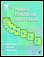Parameter Estimation and Inverse Problems, by Richard Aster, Brian Borchers, & Clifford Thurber. For beginners to inversion, I strongly recommend this book above other inverse theory textbooks; there are plenty very useful books on the topic, but this one really gets you up to speed in the subject fast with great hands-on Matlab examples. Then, after you're more familiar with the material, go back and reread the book again - there are tons of handy comparisons between methods with references to deeper treatment of the individual methods elsewhere, and many practicality details that address numerical issues and real world limitations. This book mostly focuses on frequentist inversion but does have a chapter on Bayesian inversion comparing the two approaches. This book comes with a CD with a copy of Per Christian Hansen's (linear) regularization tools for Matlab, used in most of the book's example problems, whose Matlab scripts are also on the CD. Note also the homepage for this book which includes the errata.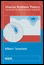Inverse Problem Theory, by Albert Tarantola. Very well written book with a probabilistic approach to inversion, in contrast with the frequentist statistical approach focused on in Aster/Borchers/Thurber, Menke, Hansen, and Parker. Tarantola doesn't like to call it "Bayesian" (even though it's essentially the same formulation), for he rederives it differently to address concerns such as Borel's paradox seen in Bayesian philosophy. In any case, this probabilistic approach allows for the same type of linearization approach often done for weakly nonlinear problems in the frequentist approach, but additionally provides for a very flexible numerical sampling approach to handle more strongly nonlinear problems. In spite of the focus on the probabilistic approach, this book has many useful comparisons between probabilistic (Bayesian) and frequentist inverse theory. Note he offers a free PDF copy of this book on his webpage (with a request to please buy the book if you find yourself using it and can afford it). For those who are familiar with his 1987 book, this one largely replaces it but leaves out a few bits I thought were really useful in the 1987 version, like expressions for derivatives of elastic displacement with respect to media properties. But many of the other example problems remain.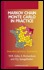Markov Chain Monte Carlo in Practice, by W.R. Gilks, S. Richardson, & D.J. Spiegelhalter. Markov Chain Monte Carlo (MCMC) sampling, among other uses, can be used for numerically sampling the posterior distribution in Bayesian (probabilistic) inversion. The book is a fantastic and rigorous supplement to Tarantola's book. The 19 page introductory chapter (16 pages without the refs) is such an accessible overview of the subject of MCMC that it would make a very good reading assignment for an inverse theory class that discussed Bayesian inversion. The rest of the chapters are articles by experts in the field, detailing implementation issues like convergence, sampling mechanics, and differences between variations of MCMC such as Metropolis/Hastings vs Gibbs sampling and so on. An important note made in the introduction is that MCMC is not only for Bayesian inversion -- in frequentist inversion one may wish to sample the integral in the data misfit distribution (say if it were some arbitrary distribution other than Gaussian).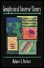Geophysical Inverse Theory, by Robert L. Parker. A classic frequentist text that is very readable - Parker is rigorous and introduces the reader to functional analysis concepts, but injects witty tidbits here and there which keep you interested. This book focuses on the Gram matrix / representers technique, which parameterizes the model with the same number of parameters as there are data points, and requires numerical integration for many real-world problems. But the technique is ideal for gravity inversion and magnetotellurics (MT), and those two topics are explored in detail in the book's examples. So you should be sure to read this book if you are working on gravity or MT inversion.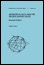Geophysical Data Analysis: Discrete Inverse Theory, Revised Edition, by William Menke. Another frequentist classic text; note the revised edition is recommended as the original had numerous typos in the equations. While Parker leaves the Earth model being estimated as a continuous function, Menke (like Aster/Borchers/Thurber and others) discretizes it as his parameterization. The idea is that this discretization is finer than the resolution of the inverse problem solution. This book is the only one in which I've seen much discussion of the data resolution matrix (as opposed to model resolution matrix).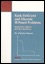Rank-Deficient and Discrete Ill-Posed Problems: Numerical Aspects of Linear Inversion, by Per Christian Hansen. Very well written book concerning various regularization methods in frequentist inversion, with emphasis on a SVD/spectral approach. The value of formulating regularization in the SVD domain is twofold -- one, it's greatly faster computationally (analogous to speeding up timeseries processing by doing it in the frequency domain), and two, it does a much better job at preserving numerical accuracy. A good chunk of this book's material (but not all) can be found in Hansen's Regularization Tools manual (below under WWW links). And that's free unlike this book!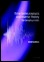Time Series Analysis and Inverse Theory for Geophysicists, by David Gubbins. As the title implies, this book is sortof two books in one, and so far it is the inverse theory half that I've spent time reading. Unfortunately I found it somewhat scattered and think it would be very difficult to learn the material for the first time from this book. On the other hand however, this book is worth reading after being more familiar with the topic, as there are useful tidbits throughout. Just as one example, this was the only inverse theory book I've found so far that specifically points out that the model resolution matrix is not always symmetric (for example when using 2nd-order Tikhonov regularization). That drove me nuts figuring that out, but wouldn't have if I'd come across this book sooner.

• A Reading List in Inverse Problems, Brian Borchers, 1998.
A very useful bibliography of inverse theory references, both classic and modern, with brief 1-2 sentence summaries.
• Resolution of seismic waveform inversion: Bayes versus Occam, Gouveia & Scales, 1997.
A fantastically helpful paper comparing and contrasting Bayesian and Occam/classical inversion techniques. Explains what happened to the regularization bias and tradeoff parameter in the Bayesian approach. (By the way, you'll find the Bayesian interpretation of the resolution matrix in Tarantola's book above.)
• Inverse Problems in Geophysics, Snieder & Trampert, 2000.
At 73 pages long, it's somewhere between an article and a book. The whole thing is well-written and useful, but if nothing else I strongly recommend the first five pages as a particularly good conceptual overview of geophysical inverse theory.
• Estimation of information content and efficiency for different data sets and inversion schemes using the generalized singular value decomposition, Th. Günther, S. Friedel & K. Spitzer, 2003.
A handy addendum to the discussion of the GSVD based inversion and resolution material in section 5.5 of the Aster et al textbook, which describes resolution for higher-order (eg 2nd) Tikhonov regularization. This paper simply clarifies the GSVD material for the case when the model norm is ||L(m-m0)||2, instead of just ||Lm||2. This comes up for example in a locally linearized (nonlinear) problem where we want to smooth the actual model rather than the model perturbation that is solved for at each iteration. This nonlinear inversion topic via the regularized, iterative Gauss-Newton method is discussed further in section 10.1 of the Aster et al book. (And that's the nonlinear method we learned in ESS523 class.) You can do the problem without the GSVD stuff, it just goes faster in GSVD (analogous to how sometimes timeseries processing is faster in frequency space - GSVD space is in fact a type of spectral space), and the GSVD filtering approach may give some more intuitive feel for stabilizing the problem.
• Regularization Tools User's Manual, Hansen, 2001.
The first half of this user's manual for this very useful software is a very well written treatise on regularization of linear inverse problems. This is a .zip file which contains a postscript file.
• Monte Carlo sampling of solutions to inverse problems, Mosegaard & Tarantola, 1995.
This and the next paper address a useful Monte Carlo technique for handling nonlinear inversions, including the non-Gaussian statistics of their solution. This is an ideal nonlinear technique when your problem is small/fast enough that you can run your forward problem many many times in a reasonable amount of computation time.
• Resolution Analysis of General Inverse Problems through Inverse Monte Carlo Sampling, Mosegaard, 1998.
• The Role of Nonlinearity in Inverse Problems, Snieder, 1998.